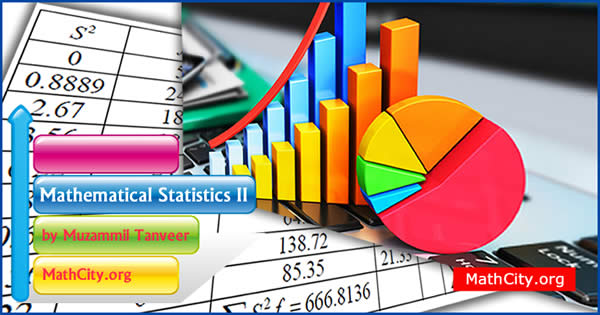# Mathematical Statistics II by Sir Haidar AliA subfield of mathematics called mathematical statistics is concerned with using mathematical techniques to solve statistical problems. It involves using mathematical analysis and probability theory to the study of statistical issues like estimate, hypothesis testing, and confidence intervals. Financial, engineering, and scientific fields all benefit from the use of mathematical statistics, which is a significant area of study in both pure and applied mathematics.

These notes are provided and composed by Mr. Muzammil Tanveer. We are really very thankful to him for providing these notes and appreciates his effort to publish these notes on MathCity.org

• Name: Mathematical Statistics II
• Provider: Mr. Muzammil Tanveer
• Lectured by: Sir Haider Ali
• Pages: 97 pages
• Format: PDF
• Size: 789 KB
• Category: Statistics
• Population
• Size of Population
• Sample
• Sampling
• Sampling Unit
• Random Sample
• Parameter
• Statistics
• Sampling Distribution
• Sampling Distribution with Replacement and without Replacement
• Statistical Inference
• Point Estimation
• Interval Estimation
• Point Estimation
• Good point Estimator
• Criteria for Good point Estimator
• Unbiasedness
• Normal Distribution Density function
• Efficiency
• Consistency
• Criteria for Consistency
• Sufficiency
• Neyman-Fisherman Criterion for sufficiency
• Methods of point estimator
• The method of maximum likelihood estimator
• Likelihood function
• Maximum likelihood Estimator
• Normal Distribution
• Moment Generating function (m.g.f)
• Properties of Normal Distribution
• Degree of freedom
• Chi-Square Random variable
• Chi-Square Distribution
• Properties of Chi-Square Distribution
• T-Distribution
• Properties of T-Distribution
• F-Distribution
• Properties of F-Distribution

Please click on View Online to see inside the PDF.

• notes/mathematical-statistics-ii-muzammil-tanveer